Parametrization of ggplot2::geom_segment either by location and displacement or by magnitude and angle with default arrows. geom_arrow() is the same as geom_vector() but defaults to preserving the direction under coordinate transformation and different plot ratios.

geom_arrow(
mapping = NULL,
data = NULL,
stat = "arrow",
position = "identity",
...,
start = 0,
direction = c("ccw", "cw"),
pivot = 0.5,
preserve.dir = TRUE,
min.mag = 0,
skip = 0,
skip.x = skip,
skip.y = skip,
arrow.angle = 15,
arrow.length = 0.5,
arrow.ends = "last",
arrow.type = "closed",
arrow = grid::arrow(arrow.angle, grid::unit(arrow.length, "lines"), ends = arrow.ends,
type = arrow.type),
lineend = "butt",
na.rm = FALSE,
show.legend = NA,
inherit.aes = TRUE
)

geom_vector(
mapping = NULL,
data = NULL,
stat = "arrow",
position = "identity",
...,
start = 0,
direction = c("ccw", "cw"),
pivot = 0.5,
preserve.dir = FALSE,
min.mag = 0,
skip = 0,
skip.x = skip,
skip.y = skip,
arrow.angle = 15,
arrow.length = 0.5,
arrow.ends = "last",
arrow.type = "closed",
arrow = grid::arrow(arrow.angle, grid::unit(arrow.length, "lines"), ends = arrow.ends,
type = arrow.type),
lineend = "butt",
na.rm = FALSE,
show.legend = NA,
inherit.aes = TRUE
)

## Arguments

mapping

Set of aesthetic mappings created by aes(). If specified and inherit.aes = TRUE (the default), it is combined with the default mapping at the top level of the plot. You must supply mapping if there is no plot mapping.

data

The data to be displayed in this layer. There are three options:

If NULL, the default, the data is inherited from the plot data as specified in the call to ggplot().

A data.frame, or other object, will override the plot data. All objects will be fortified to produce a data frame. See fortify() for which variables will be created.

A function will be called with a single argument, the plot data. The return value must be a data.frame, and will be used as the layer data. A function can be created from a formula (e.g. ~ head(.x, 10)).

stat

The statistical transformation to use on the data for this layer, either as a ggproto Geom subclass or as a string naming the stat stripped of the stat_ prefix (e.g. "count" rather than "stat_count")

position

Position adjustment, either as a string naming the adjustment (e.g. "jitter" to use position_jitter), or the result of a call to a position adjustment function. Use the latter if you need to change the settings of the adjustment.

...

Other arguments passed on to layer(). These are often aesthetics, used to set an aesthetic to a fixed value, like colour = "red" or size = 3. They may also be parameters to the paired geom/stat.

start

starting angle for rotation in degrees

direction

direction of rotation (counter-clockwise or clockwise)

pivot

numeric indicating where to pivot the arrow where 0 means at the beginning and 1 means at the end.

preserve.dir

logical indicating whether to preserve direction or not

min.mag

minimum magnitude for plotting vectors

skip, skip.x, skip.y

numeric specifying number of gridpoints not to draw in the x and y direction

arrow.length, arrow.angle, arrow.ends, arrow.type

parameters passed to grid::arrow

arrow

specification for arrow heads, as created by grid::arrow().

lineend

Line end style (round, butt, square).

na.rm

If FALSE, the default, missing values are removed with a warning. If TRUE, missing values are silently removed.

show.legend

logical. Should this layer be included in the legends? NA, the default, includes if any aesthetics are mapped. FALSE never includes, and TRUE always includes. It can also be a named logical vector to finely select the aesthetics to display.

inherit.aes

If FALSE, overrides the default aesthetics, rather than combining with them. This is most useful for helper functions that define both data and aesthetics and shouldn't inherit behaviour from the default plot specification, e.g. borders().

## Details

Direction and start allows to work with different standards. For the meteorological standard, for example, use star = -90 and direction = "cw".

## Aesthetics

geom_vector understands the following aesthetics (required aesthetics are in bold)

• x

• y

• either mag and angle, or dx and dy

• alpha

• colour

• linetype

• size

• lineend

Other ggplot2 helpers: MakeBreaks(), WrapCircular(), geom_contour2(), geom_contour_fill(), geom_label_contour(), geom_relief(), geom_streamline(), guide_colourstrip(), map_labels, reverselog_trans(), scale_divergent, scale_longitude, stat_na(), stat_subset()

## Examples

library(data.table)
library(ggplot2)

data(seals)
# If the velocity components are in the same units as the axis,
# geom_vector() (or geom_arrow(preserve.dir = TRUE)) might be a better option
ggplot(seals, aes(long, lat)) +
geom_arrow(aes(dx = delta_long, dy = delta_lat), skip = 1, color = "red") +
geom_vector(aes(dx = delta_long, dy = delta_lat), skip = 1) +
scale_mag()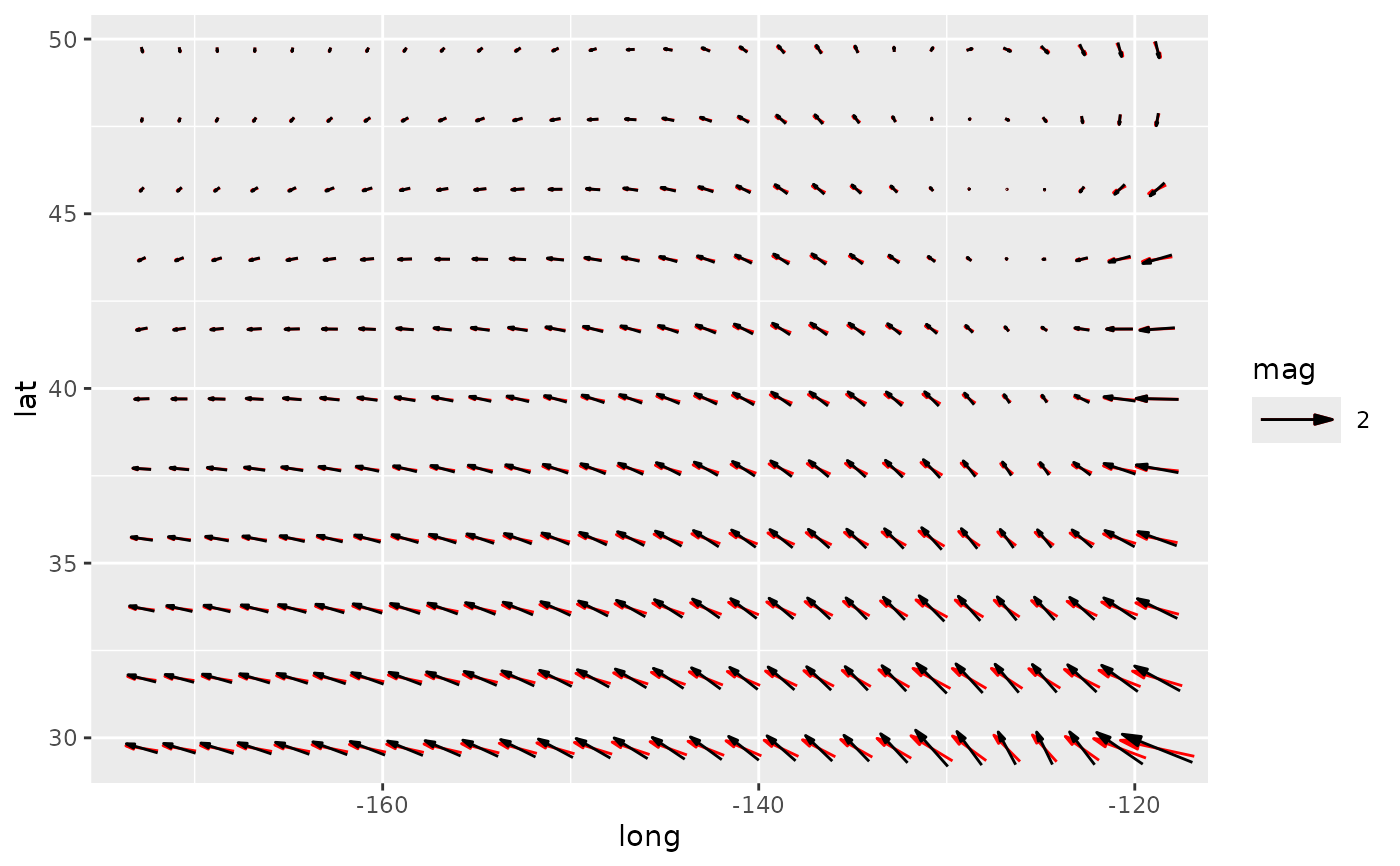data(geopotential)
geopotential <- copy(geopotential)[date == date]
geopotential[, gh.z := Anomaly(gh), by = .(lat)]
#>         lon   lat lev       gh       date     gh.z
#>    1:   0.0 -22.5 700 3163.839 1990-01-01 13.67219
#>    2:   2.5 -22.5 700 3162.516 1990-01-01 12.34968
#>    3:   5.0 -22.5 700 3162.226 1990-01-01 12.05939
#>    4:   7.5 -22.5 700 3162.323 1990-01-01 12.15607
#>    5:  10.0 -22.5 700 3163.097 1990-01-01 12.93024
#>   ---
#> 4028: 347.5 -90.0 700 2715.936 1990-01-01  0.00000
#> 4029: 350.0 -90.0 700 2715.936 1990-01-01  0.00000
#> 4030: 352.5 -90.0 700 2715.936 1990-01-01  0.00000
#> 4031: 355.0 -90.0 700 2715.936 1990-01-01  0.00000
#> 4032: 357.5 -90.0 700 2715.936 1990-01-01  0.00000
geopotential[, c("u", "v") := GeostrophicWind(gh.z, lon, lat)]
#>         lon   lat lev       gh       date     gh.z  u           v
#>    1:   0.0 -22.5 700 3163.839 1990-01-01 13.67219 NA  1.08181190
#>    2:   2.5 -22.5 700 3162.516 1990-01-01 12.34968 NA  0.55189199
#>    3:   5.0 -22.5 700 3162.226 1990-01-01 12.05939 NA  0.06625043
#>    4:   7.5 -22.5 700 3162.323 1990-01-01 12.15607 NA -0.29800162
#>    5:  10.0 -22.5 700 3163.097 1990-01-01 12.93024 NA -0.75064329
#>   ---
#> 4028: 347.5 -90.0 700 2715.936 1990-01-01  0.00000 NA  0.00000000
#> 4029: 350.0 -90.0 700 2715.936 1990-01-01  0.00000 NA  0.00000000
#> 4030: 352.5 -90.0 700 2715.936 1990-01-01  0.00000 NA  0.00000000
#> 4031: 355.0 -90.0 700 2715.936 1990-01-01  0.00000 NA  0.00000000
#> 4032: 357.5 -90.0 700 2715.936 1990-01-01  0.00000 NA  0.00000000

(g <- ggplot(geopotential, aes(lon, lat)) +
geom_arrow(aes(dx = dlon(u, lat), dy = dlat(v)), skip.x = 3, skip.y = 2,
color = "red") +
geom_vector(aes(dx = dlon(u, lat), dy = dlat(v)), skip.x = 3, skip.y = 2) +
scale_mag(max_size = 2, guide = "none"))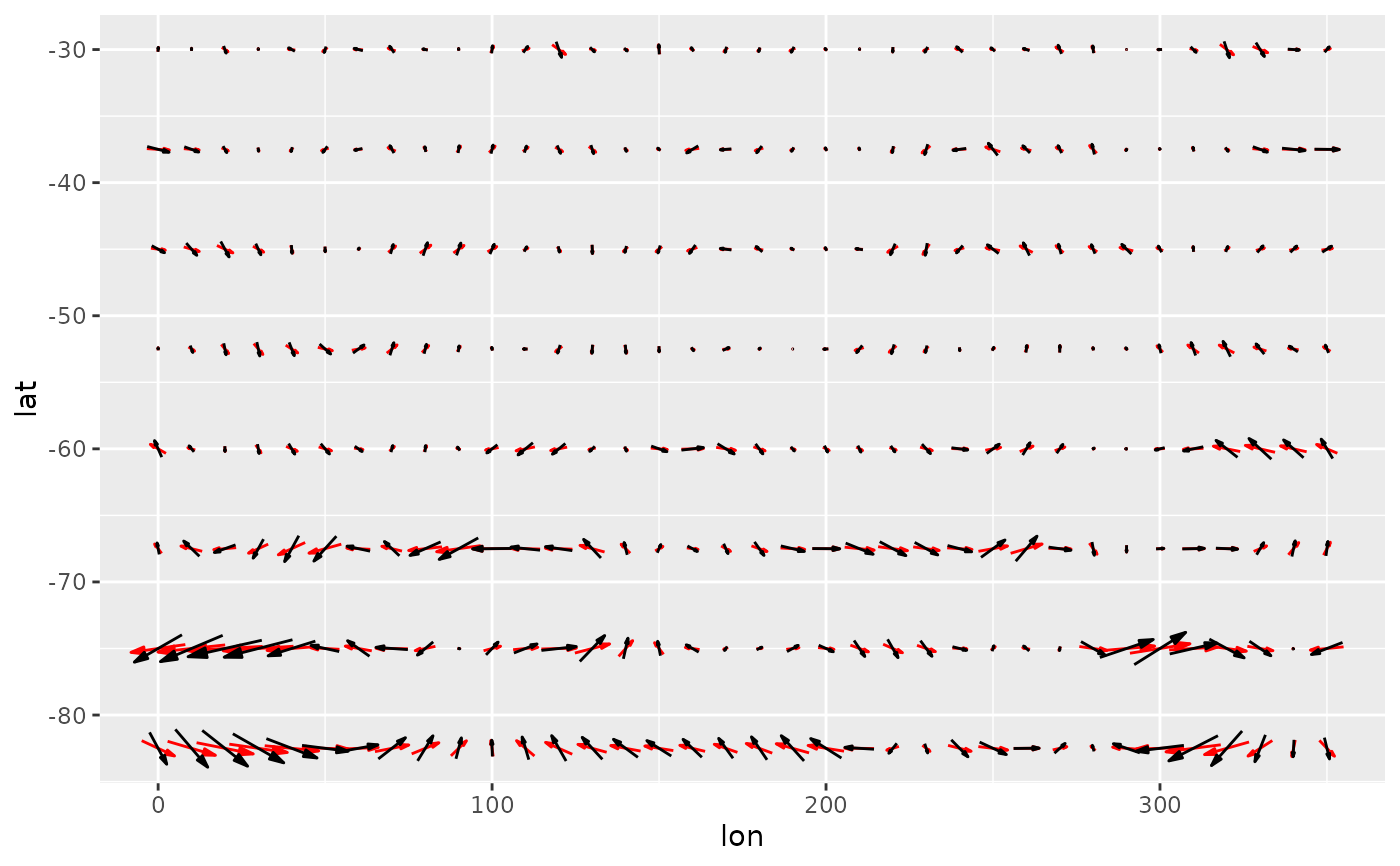# A dramatic illustration of the difference between arrow and vector
g + coord_polar()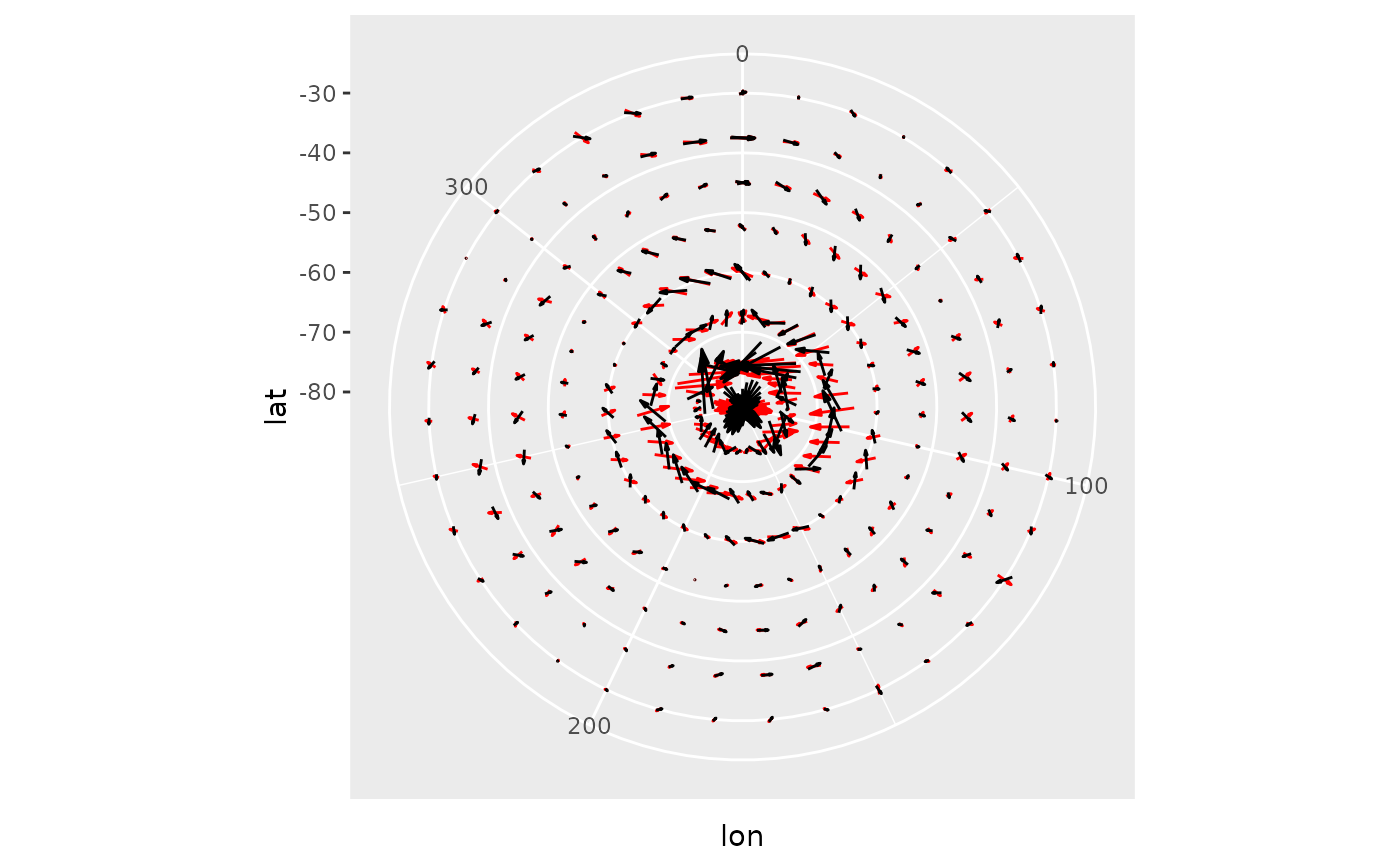# When plotting winds in a lat-lon grid, a good way to have both
# the correct direction and an interpretable magnitude is to define
# the angle by the longitud and latitude displacement and the magnitude
# by the wind velocity. That way arrows are always parallel to streamlines
# and their magnitude are in the correct units.
ggplot(geopotential, aes(lon, lat)) +
geom_contour(aes(z = gh.z)) +
geom_vector(aes(angle = atan2(dlat(v), dlon(u, lat))*180/pi,
mag = Mag(v, u)), skip = 1, pivot = 0.5) +
scale_mag()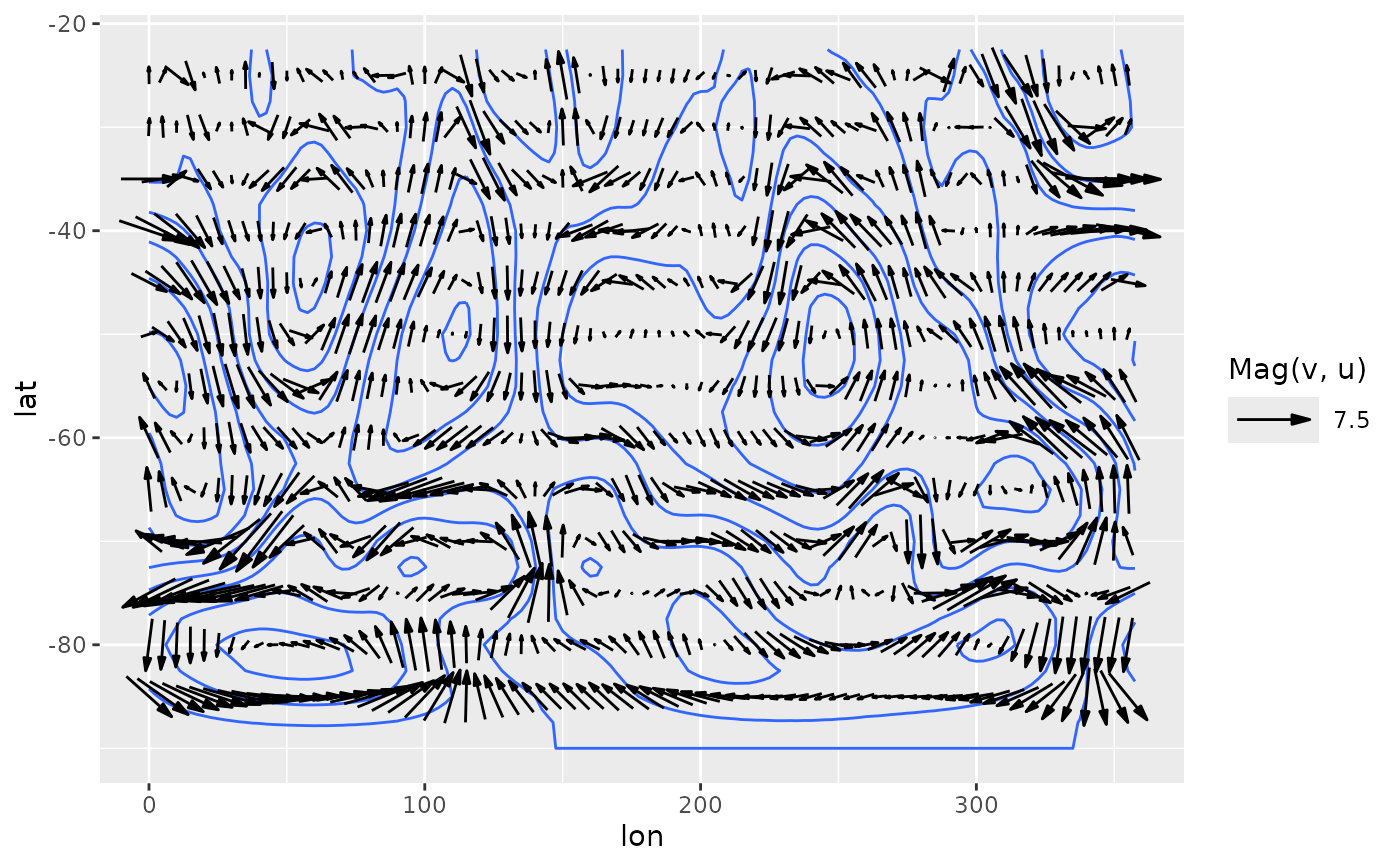# Sverdrup transport
library(data.table)
b <- 10
d <- 10
grid <- as.data.table(expand.grid(x = seq(1, d, by = 0.5),
y = seq(1, b, by = 0.5)))
grid[, My := -sin(pi*y/b)*pi/b]
#>         x  y            My
#>   1:  1.0  1 -9.708055e-02
#>   2:  1.5  1 -9.708055e-02
#>   3:  2.0  1 -9.708055e-02
#>   4:  2.5  1 -9.708055e-02
#>   5:  3.0  1 -9.708055e-02
#>  ---
#> 357:  8.0 10 -3.847341e-17
#> 358:  8.5 10 -3.847341e-17
#> 359:  9.0 10 -3.847341e-17
#> 360:  9.5 10 -3.847341e-17
#> 361: 10.0 10 -3.847341e-17
grid[, Mx := -pi^2/b^2*cos(pi*y/b)*(d - x)]
#>         x  y            My          Mx
#>   1:  1.0  1 -9.708055e-02 -0.84478964
#>   2:  1.5  1 -9.708055e-02 -0.79785688
#>   3:  2.0  1 -9.708055e-02 -0.75092413
#>   4:  2.5  1 -9.708055e-02 -0.70399137
#>   5:  3.0  1 -9.708055e-02 -0.65705861
#>  ---
#> 357:  8.0 10 -3.847341e-17  0.19739209
#> 358:  8.5 10 -3.847341e-17  0.14804407
#> 359:  9.0 10 -3.847341e-17  0.09869604
#> 360:  9.5 10 -3.847341e-17  0.04934802
#> 361: 10.0 10 -3.847341e-17  0.00000000

ggplot(grid, aes(x, y)) +
geom_arrow(aes(dx = Mx, dy = My))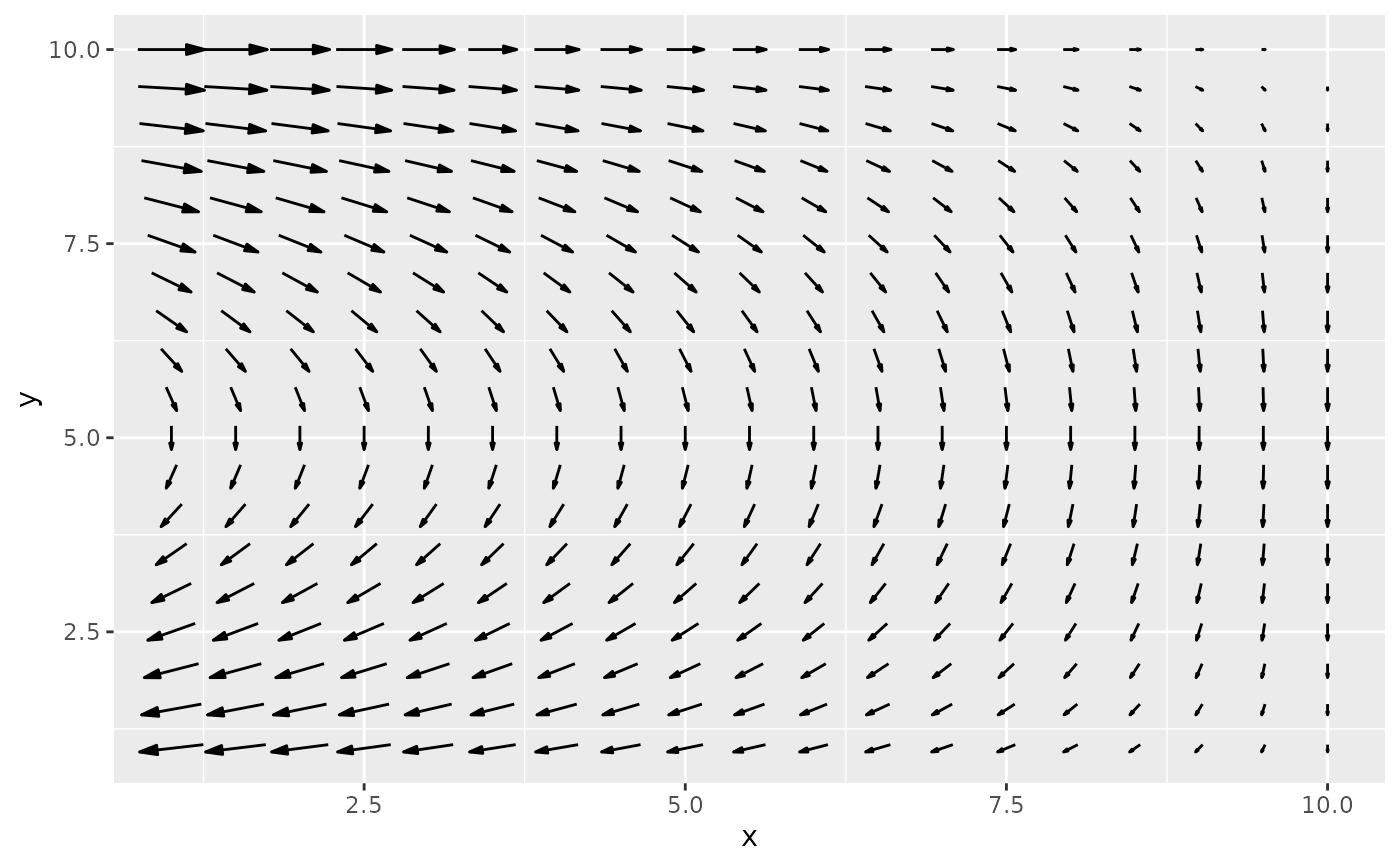# Due to limitations in ggplot2 (see: https://github.com/tidyverse/ggplot2/issues/4291),
# if you define the vector with the dx and dy aesthetics, you need
# to explicitly add scale_mag() in order to show the arrow legend.

ggplot(grid, aes(x, y)) +
geom_arrow(aes(dx = Mx, dy = My)) +
scale_mag()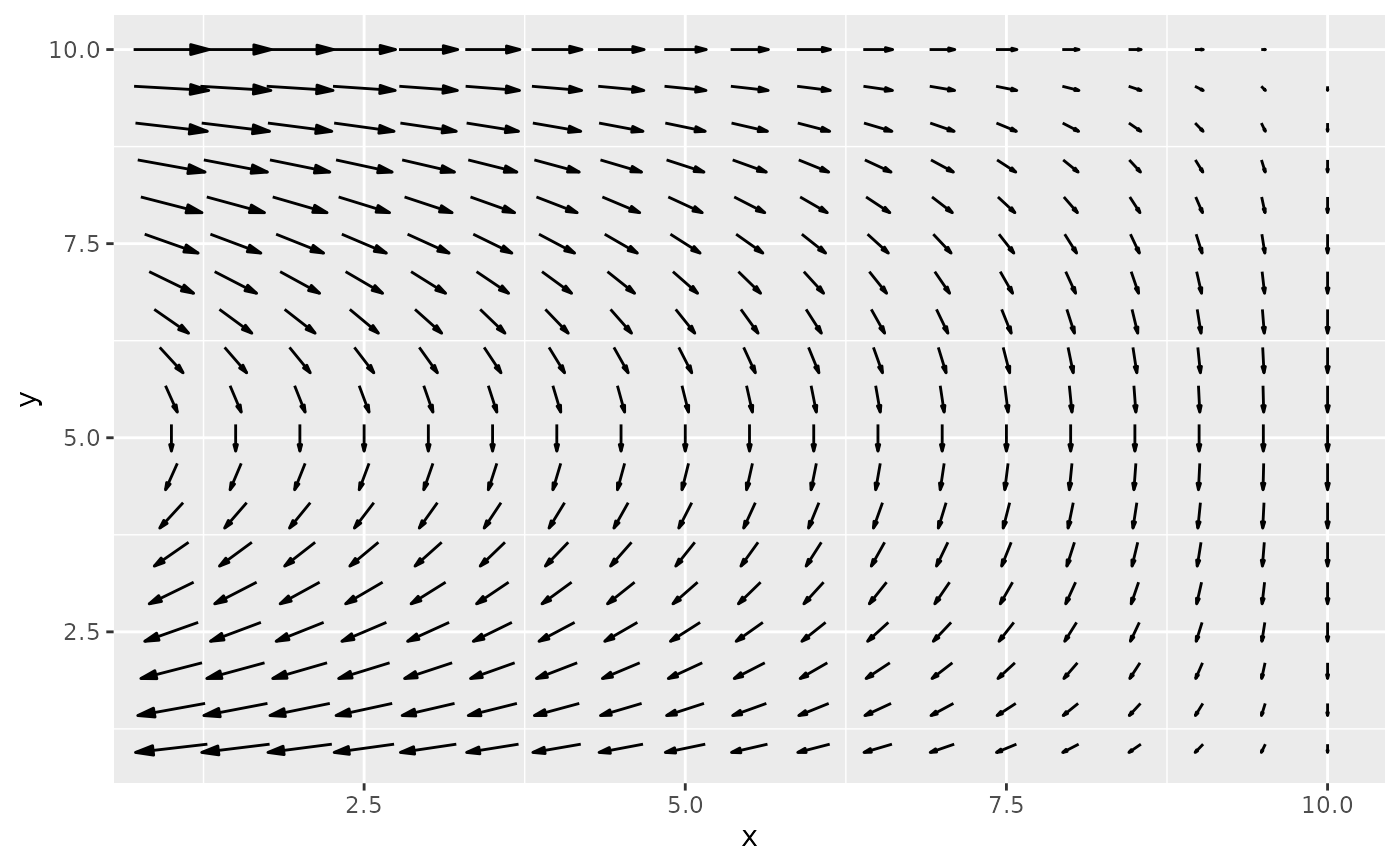# Alternative, use Mag and Angle.
ggplot(grid, aes(x, y)) +
geom_arrow(aes(mag = Mag(Mx, My), angle = Angle(Mx, My)))